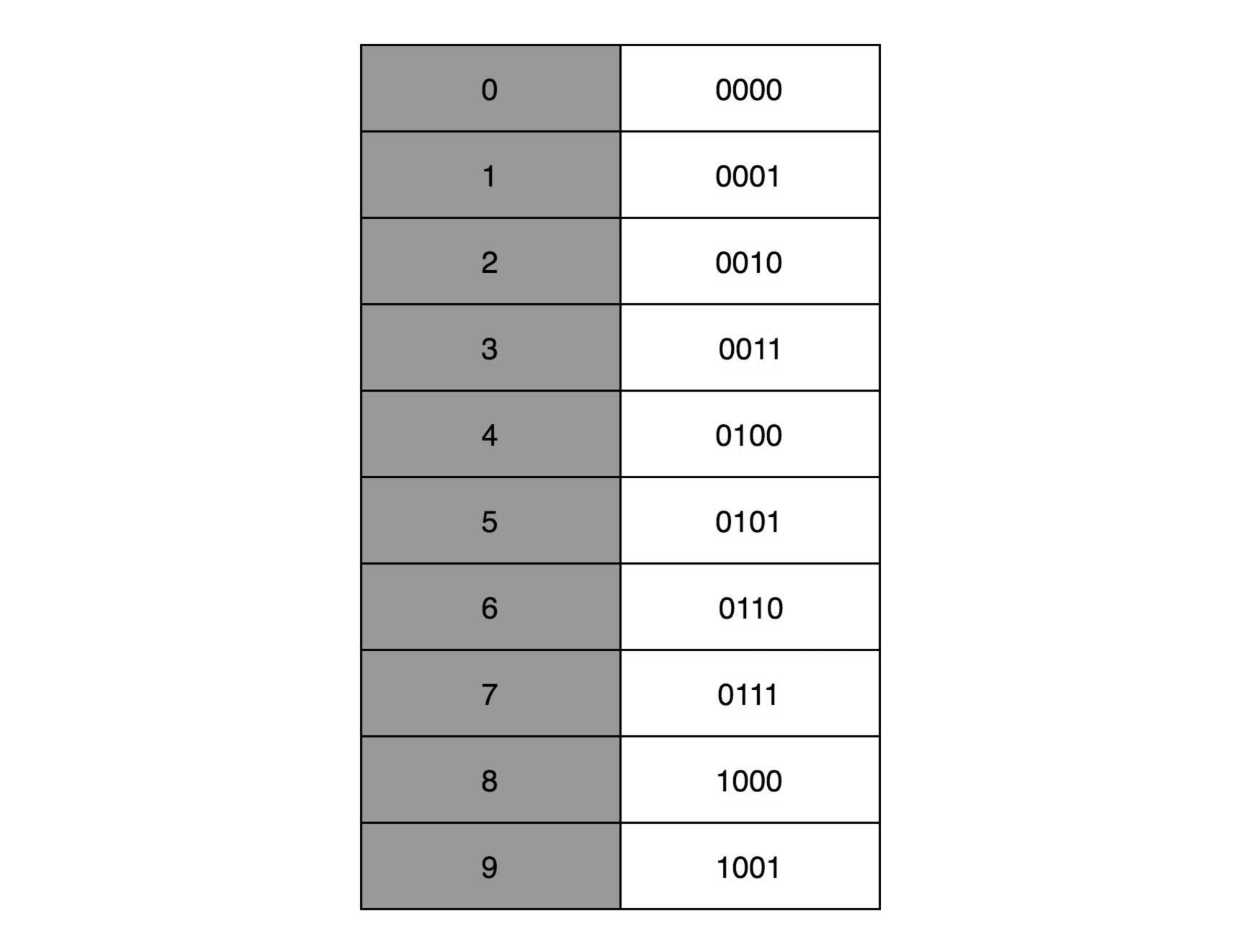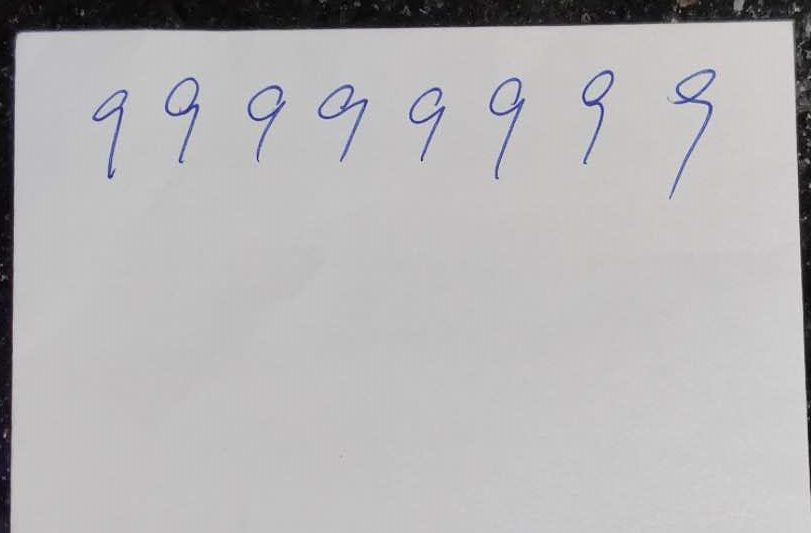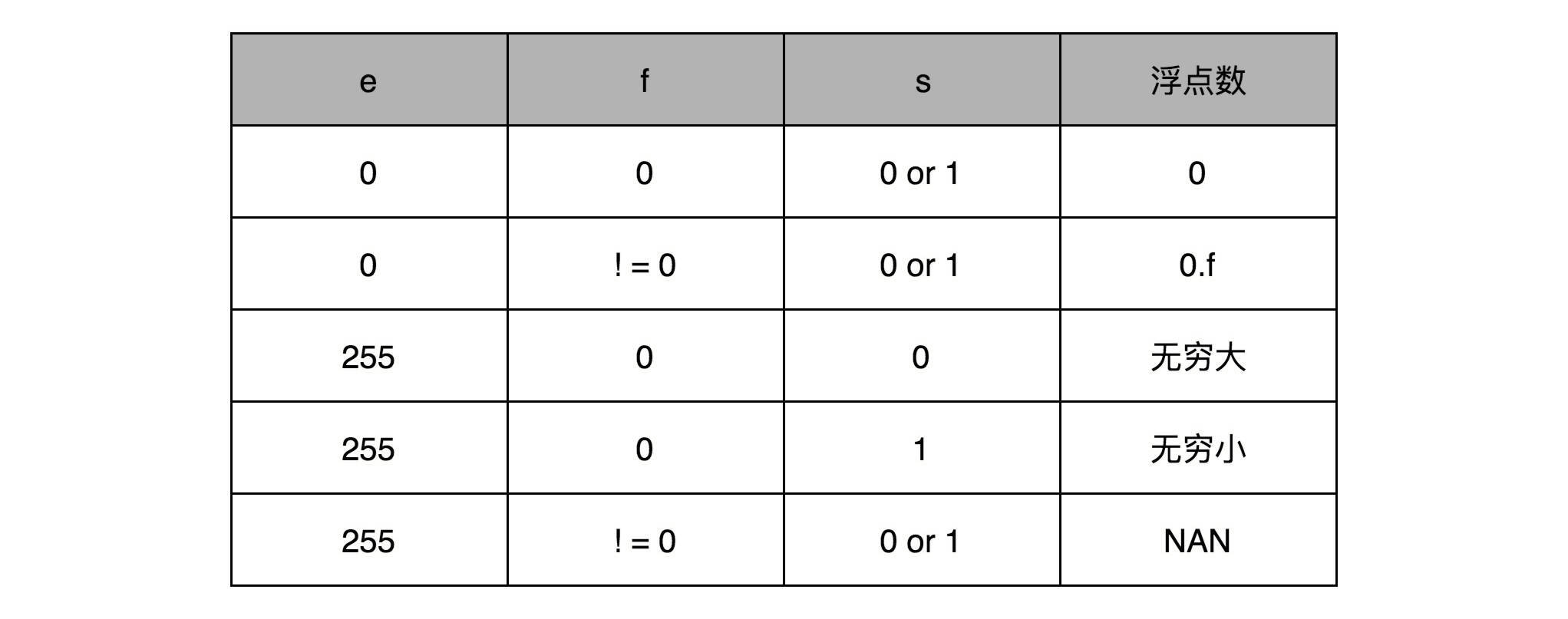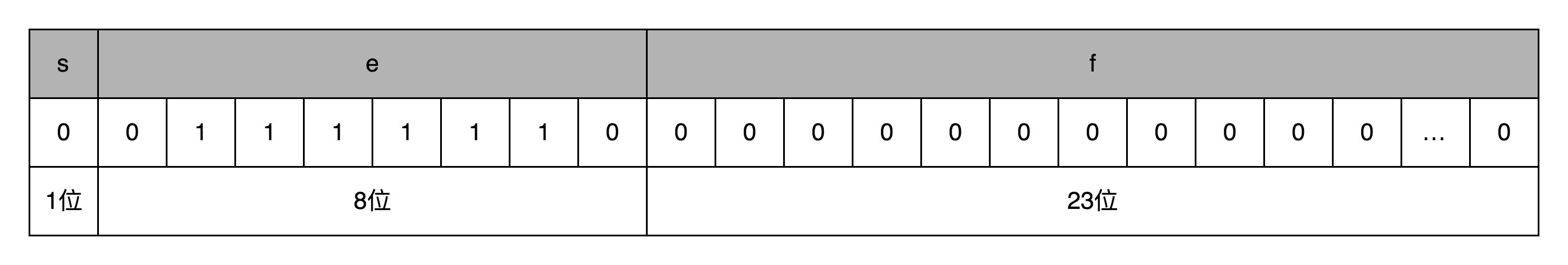15 浮点数和定点数（上）：怎么用有限的Bit表示尽可能多的信息？

## 浮点数的不精确性

``````>>> 0.3 + 0.6
0.8999999999999999
``````

40 亿个数看似已经很多了，但是比起无限多的实数集合却只是沧海一粟。所以，这个时候，计算机的设计者们，就要面临一个问题了：我到底应该让这 40 亿个数映射到实数集合上的哪些数，在实际应用中才能最划得来呢？

## 定点数的表示## 浮点数的表示(−1)s×1.f×2e(−1)s×1.f×2e0.5=(−1)0×1.0×2−1=0.50.5=(−1)0×1.0×2−1=0.5，对应的浮点数表示，就是 32 个比特。s=0，e=2−1s=0，e=2−1，需要注意，e 表示从 -126 到 127 个，-1 是其中的第 126 个数，这里的 e 如果用整数表示，就是 26+25+24+23+22+21=12626+25+24+23+22+21=126，1.f=1.01.f=1.0。